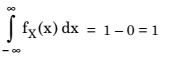# Probability Density Function (PDF)

## Probability Density Function (PDF)

The cumulative distribution function (CDF) can give useful information about discrete as well as continuous random variables. However, the probability density function (PDF) is a more convenient way of describing a continuous random variable. The probability density function fX(x) is defined as the derivative of the cumulative distribution function. Thus, we have,### Properties of PDF

Property 1: The CDF can be derived from PDF by integrating it i.e.,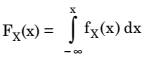Proof :

According to the definition of PDF , we haveIntegrating both the sides, we getIt is important to note the upper limit of integration. It is not + ∞ but, it is ‘x’. This is because FX(x) has been defined as the probability of X ≤ x.

Thus,But, FX(– ∞) = 0

Therefore,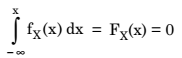or,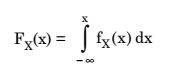Property 2 : PDF is a non-negative function for all values of x i.e.,

fX(x) ≥ 0      for all x

Reasoning: As we know that CDF is a monotone increasing function. PDF is the derivative of CDF and the derivative of a monotone increasing function will always be positive.

Property 3: The area under PDF curve is always equal to unity.

Therefore,Proof :

As per the definition of PDF, we have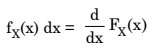Integrating both sides, we get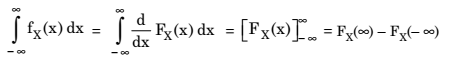But,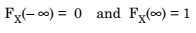Therefore,